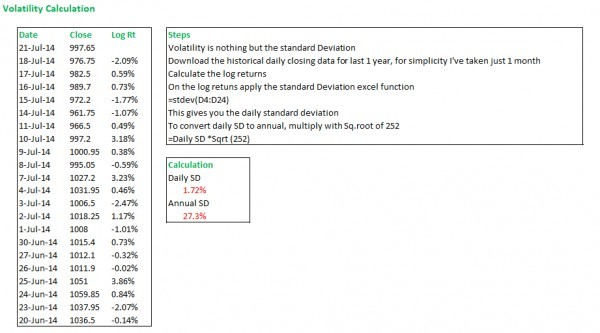# How do we measure volatility for stock or share?

1 Like

u can use ATR to measure the volatility…

1 Like

I'm assuming you are asking about a historical volatility. I've used Reliance as an example..6 Likes

How can I calculate the volatility using similar method for odd number of days like day past 10 days or 50 days.
Thanks

For any time period just multiply the daily vol with sqrt of time. For example for 10 days it would be 1,72%*Sqrt(10). For 50 days it would be 1.72%*sqrt(50).

Very beautiful explanation sir…but how to make calculations for log return?

It’s Simple to Calcuate Log Return Just Take the Latter Price i.e 976.75 and subtract the former Day Price i.e 997.65 and divide the result by 997.65 (Former Price) 100 you will get the Log Return as -2.09%.